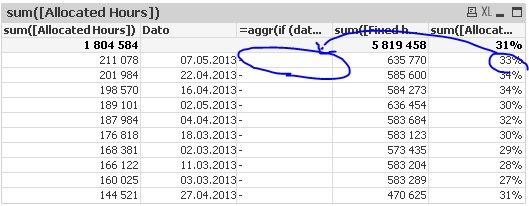# QlikView Creating Analytics

Discussion Board for collaboration related to Creating Analytics for QlikView.

HighlightedNot applicable

## Calculating on a subset of a table

Hi guys!

My first post here, problaly not the last. And hope to contribute in a whileConsider this table:I want to calculate and show the Percentage only for the latest (max(Dato)) date. How can I accomplish this?

Best regards

Trond Erik

Tags (3)
1 Solution

Accepted Solutions
Contributor III

## Re: Calculating on a subset of a table

If(Date=Max(Total Date), Expression ))

3 Replies
Contributor III

## Re: Calculating on a subset of a table

If(Date=Max(Total Date), Expression ))Not applicable

## Re: Calculating on a subset of a table

As an expression or calculated dimension?

Contributor III

## Re: Calculating on a subset of a table

As an expression.